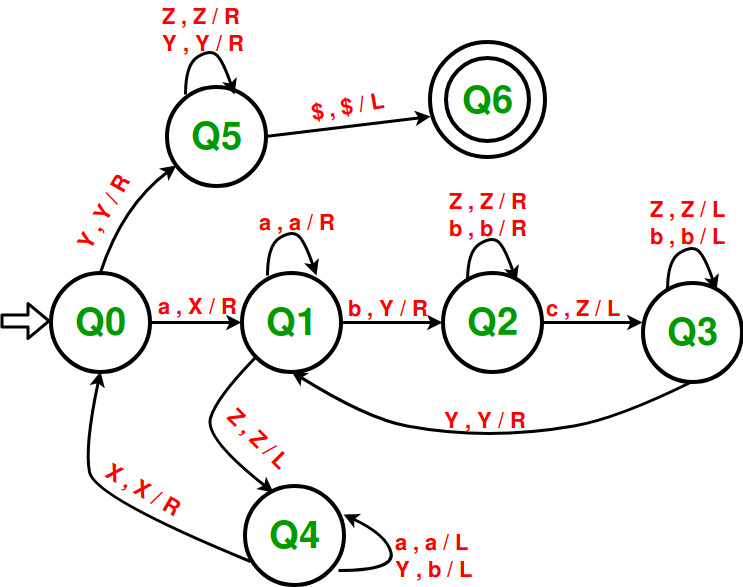# Construct a Turing machine for L = {aibjck | i*j = k; i, j, k ≥ 1}

Prerequisite – Turing Machine
In given language L = {aibjck | i*j = k; i, j, k ≥ 1}, every string of ‘a’, ‘b’ and ‘c’ have certain number of a’s, then certain number of b’s and then certain number of c’s. The condition is that count of each of these 3 symbols should be atleast 1. ‘a’ and ‘b’ can have thereafter be as many but count of c is equal to the product of count of ‘a’ and count of ‘b’. Assume that string is ending with ‘\$’.

Examples –

```Input: a a b b b c c c c c c
Here a = 2, b = 3, c = 2 * 3 = 6
Output: ACCEPTED

Input: a a b b c c c
Here a = 2, b = 2, c = 3 but c should be 4
Output: NOT ACCEPTED ```

Approach used – Scan the input from left.

1. First replace an ‘a’ with ‘X’ and move 1 step right. Then skip all the a’s and move right.
2. When pointer reach to the first ‘b’ stop. Replace one ‘b’ with ‘Y’, then move right skipping all intermediate b’s and corresponding to the replaced ‘b’ now replace one ‘c’ with ‘Z’ and move left.
3. Now move towards left skipping all ‘Z’ and ‘b’ in the way.
4. When pointer reach the most recent ‘Y’ move right.
5. If the pointer is pointing at ‘b’ then repeat steps 2 to 4, else if pointer is pointing at ‘Z’ then move towards left while replacing all ‘Y’ to ‘b’ and skipping all a’s.
6. When pointer comes to most recent ‘X’ move one step right.
7. If pointer is still pointing to ‘a’ then repeat all above steps, else if pointer is at ‘Y’ then move towards right skipping all ‘Y’ and ‘Z’.
8. When \$ is reached then move left. String is ACCEPTED.My Personal Notes arrow_drop_upCheck out this Author's contributed articles.

If you like GeeksforGeeks and would like to contribute, you can also write an article using contribute.geeksforgeeks.org or mail your article to contribute@geeksforgeeks.org. See your article appearing on the GeeksforGeeks main page and help other Geeks.

Please Improve this article if you find anything incorrect by clicking on the "Improve Article" button below.

Article Tags :

1

Please write to us at contribute@geeksforgeeks.org to report any issue with the above content.